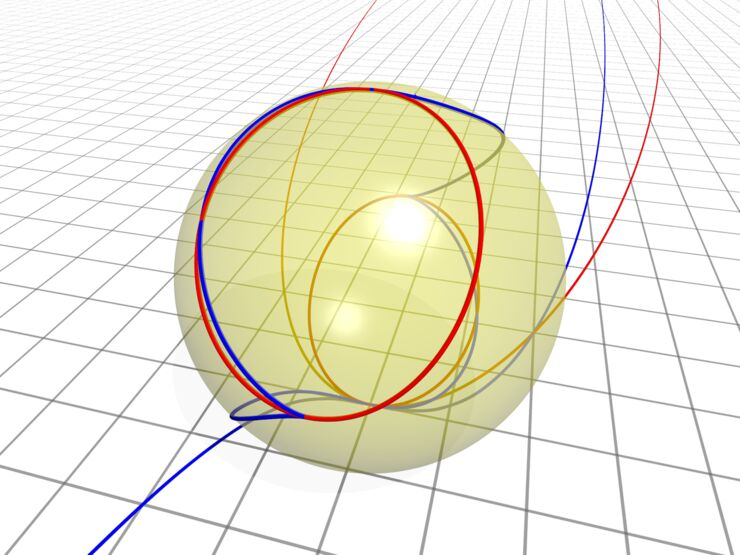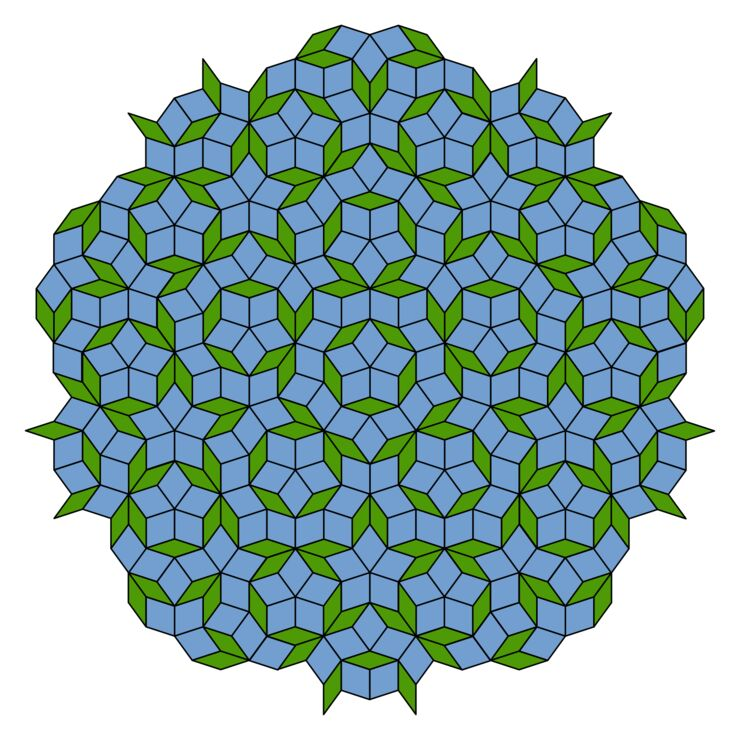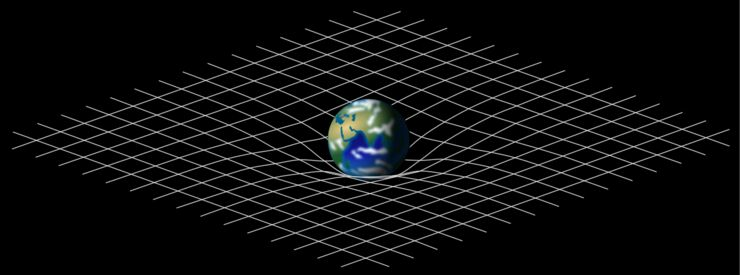Home

# Noncommutative Riemannian Geometry

The research group is focused on questions related to metric aspects of noncommutative geometry, such as Levi-Civita connections and curvature, mainly from the viewpoint of (twisted) derivation based calculi.

## Geometry from an algebraic viewpointWe are accustomed to think of geometric objects as a concrete subsets of three-dimensional space, such as lines, discs and spheres. These objects are usually studied as a collection of points for which one may introduce more or less advanced tools in order to discover properties and relations among them. For instance, one can measure
the distance between two points or study how much a curve bends as we follow it through space.

There is a, perhaps less intuitive, complementary picture, in which one focuses on functions from the object to the real (or complex) numbers, rather than the object itself. It may be surprising that, in many cases, knowing all such functions completely determines the originial geometric object. The collection of these functions is an algebra (i.e. one may add and multiply two functions and get a new function), and it actually possible to formulate our usual concepts of geometry in a purely algebraic way.

This opens up for a more abstract way of thinking of geometry. In fact, what happens if one does not care about if the algebra at hand comes from a geometric object or not? Can one still do "geometry"? Turning the question around, is it possible to attach a geometric object to every algebra? During the 20th century, the field of algebraic geometry has studied these questions and developed a powerful machinery to handle algebraic aspects of geometry.

## Noncommutative geometryA Penrose tiling with rhombi exhibiting fivefold symmetry.It may happen that one finds a geometric object for which there are extremely few interesting functions. In fact, too few to say anything useful. However, it turns out that if we allow the values of the functions to be operators, rather that numbers, a variety of interesting functions presents itself. This trick certainly comes with a price: multiplication of functions is no longer commutative. That is, since the result of applying two operators is dependent on the order in which the are applied, the order of multiplication of operator-valued functions is important. Thus, the result is that one have to try to understand the geometry of a non-commutative algebra; a field which is known as non-commutative geometry. Surprisingly, at least from a naive point of view, it is possible to formulate many concepts of geometry also for non-commutative algebras. In particular, a far reaching and powerful generalization of topology, in the context of C*-algebras, has bee carried out with applications in many fields of mathematics.

## Quantum GravityDeformation of spacetime caused by a planetary mass.Noncommutative geometry has a central role in approaching a theory of quantum gravity - the holy grail of theoretical physics. Ever since the introduction of quantum mechanics (describing the fundamental forces) and general relativity (describing the gravitational force) more than a hundred years ago, great efforts have been made to combine the two theories into a quantum theory of gravity. Although there is no satisfactory theory of quantum gravity so far, most physicists believe that spacetime itself becomes noncommutative at very small scales. Hence, to understand the nature of quantum spacetime one needs to study noncommutative geometry.

## Riemannian geometry

In general relativity, the theory of gravitation is described in terms of a metric on spacetime. Hence, the mathematics underlying the theory is differential geometry, and more specifically Riemannian geometry. If spacetimes is truly noncommutative, any theory of quantum gravity requires an understanding of metric aspects of noncommutative geometry.

Do you want to know more about noncommutative geometry? Are you
interested in writing a master or bachelor thesis? Are you considering

## Popular science talk

### Popular science talk

Popular science talk on noncommutative geometry and quantum gravity in Swedish, recorded by UR samtiden during the popular science week at LiU 2019.

## Swedish Network for Algebra and Geometry (SNAG)

### Swedish Network for Algebra and Geometry (SNAG)

The purpose of the network is to develop the interaction between mathematicians working in the fields of algebra and geometry at Swedish universities. In particular, we encourage an active participation of PhD students and young researchers with the aim to build future networks and collaborations.

The network was initiated by Joakim Arnlind (LiU), Johan Öinert (BTH) and Sergei Silvestrov (MDH) in 2018 and organizes a yearly workshop where senior researchers, as well as PhD students, present and discuss their results.